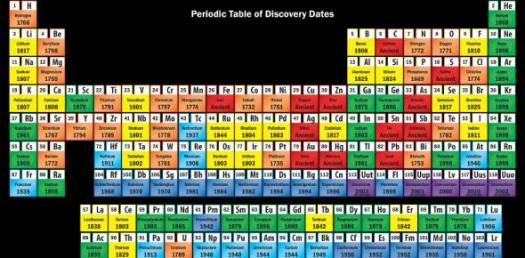# Periodic Table Mutilple Choice Quiz

24 Questions | Attempts: 375
ShareSettingsThis quiz is made up of 23 mutilple choice questions and 1 fill in the blank question. All questions are related with the periodic table.

• 1.
How many element groups are there?
• A.

7

• B.

11

• C.

16

• D.

8

• E.

26

• 2.
Who is the creator of the periodic table?
• A.

Dimitri Mendeleev

• B.

Henry Moseley

• 3.
_______________discovered the number of protons in the nucleus of each atom.
• 4.
The symbol au stands for what element?
• A.

Gold

• B.

Silver

• C.

Coper

• D.

Tin

• 5.
What is the atomic number for helium?
• A.

1

• B.

2

• C.

4

• D.

8

• 6.
What is the symbol for tin?
• A.

Sn

• B.

Se

• C.

Sg

• D.

Sr

• 7.
What is the atomic number for the element tantalum?
• A.

22

• B.

81

• C.

52

• D.

73

• 8.
What is the symbole for astatine?
• A.

As

• B.

Ai

• C.

At

• D.

Ag

• 9.
What is the atomic number for beryllium?
• A.

83

• B.

4

• C.

35

• D.

5

• 10.
The element germanium is in what element group?
• A.

Basic Metal

• B.

Non-Metal

• C.

Rare Earth

• D.

Semi-Metal

• 11.
The element Rubidium is in what element group?
• A.

Alkaline earth

• B.

Basic metal

• C.

Alkali metal

• D.

Transition metal

• 12.
What is the atomic number for cesium?
• A.

20

• B.

48

• C.

6

• D.

55

• 13.
What is the symbol for ruthenium?
• A.

Rn

• B.

Ru

• C.

Rh

• D.

Rf

• 14.
What is the symbol for manganese?
• A.

Mo

• B.

Mg

• C.

Mt

• D.

Mn

• 15.
What is the symbol for phosphorus?
• A.

Po

• B.

P

• C.

Pb

• D.

Pu

• 16.
The symbol Fe stands for which element?
• A.

Francium

• B.

Fluorine

• C.

Iodine

• D.

Iron

• 17.
The element neon is from what group?
• A.

Halogen

• B.

Rare Earth

• C.

Noble Gas

• D.

Non-Metal

• 18.
What is the atomic number of damstadtium?
• A.

105

• B.

55

• C.

110

• D.

54

• 19.
What is the atomic weight of copper?
• A.

58.9332

• B.

63.546

• C.

51.9961

• D.

40.078

• 20.
What is the atomic weight for Thulium?
• A.

168.93421

• B.

98.9072

• C.

204.3833

• D.

127.6

• 21.
What is the atomic number for mercury?
• A.

108

• B.

25

• C.

80

• D.

34

• 22.
Which element is the most abundant element in the universe?
• A.

Oxygen

• B.

Silicon

• C.

Hydrogen

• D.

Hellium

• 23.
Francium is the most rare element?
• A.

True

• B.

False

• 24.
What is the atomic number for the element Zirconium?
• A.

116

• B.

23

• C.

107

• D.

40

## Related TopicsBack to top
×

Wait!
Here's an interesting quiz for you.